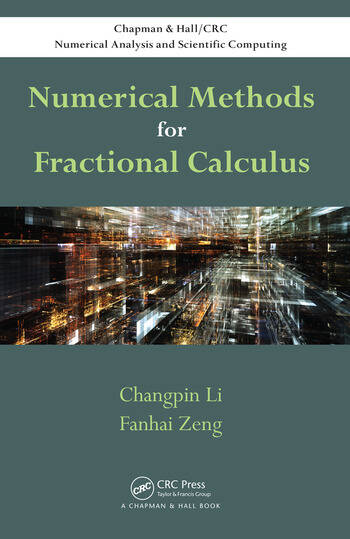Numerical Methods for Fractional Calculus

1st Edition

Changpin Li, Fanhai Zeng

Chapman and Hall/CRC
Published May 19, 2015
Reference - 300 Pages - 12 B/W Illustrations
ISBN 9781482253801 - CAT# K23934
Series: Chapman & Hall/CRC Numerical Analysis and Scientific Computing Series

For Instructors Request Inspection Copy

USD\$120.00

Currently out of stock
FREE Standard Shipping!

Preview

Summary

Numerical Methods for Fractional Calculus presents numerical methods for fractional integrals and fractional derivatives, finite difference methods for fractional ordinary differential equations (FODEs) and fractional partial differential equations (FPDEs), and finite element methods for FPDEs.

The book introduces the basic definitions and properties of fractional integrals and derivatives before covering numerical methods for fractional integrals and derivatives. It then discusses finite difference methods for both FODEs and FPDEs, including the Euler and linear multistep methods. The final chapter shows how to solve FPDEs by using the finite element method.

This book provides efficient and reliable numerical methods for solving fractional calculus problems. It offers a primer for readers to further develop cutting-edge research in numerical fractional calculus. MATLAB® functions are available on the book’s CRC Press web page.

Instructors

We provide complimentary e-inspection copies of primary textbooks to instructors considering our books for course adoption.